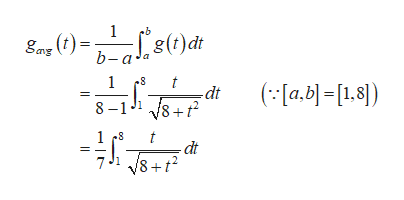Find the average value gave of the function g on the given interval.g(t)= t/sqrt(8+t2),    [1,8]

Question

Find the average value gave of the function g on the given interval.

g(t)= t/sqrt(8+t2),    [1,8]

Step 1

Obtain the average value of the function g on the g...help_outlineImage Transcriptionclose1 b g(t)dt gas b-a' 1 (a.b[1.8]) -dt 8 8-1 1 t dt 8 fullscreen

Want to see the full answer?

See Solution

Want to see this answer and more?

Our solutions are written by experts, many with advanced degrees, and available 24/7

See Solution
Tagged in

Integration